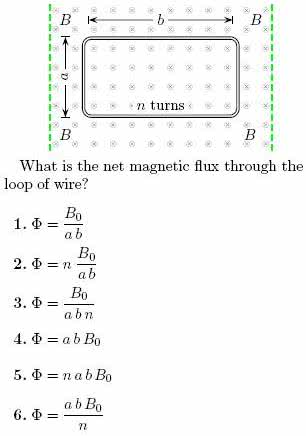1
0
watching
136
views12 Nov 2019

# (part 1 of 3)A rectangular loop of copper wire of re-sistance R has width a and length b. Theloop is stationary in a constant, uniform mag-netic field B0, directed into the page as shownabove.(part 2 of 3) Let : n = 9 , a= 9 m, b= 7 m, and B0 = 2 T. What is the induced emf inthe loop of wire? 1.E = 120 V 2.E = 1134 V 3.E = 2268 V 4.E = 240 V 5.E = 1440 V 6.E = 0 V (part 3 of 3) What is the net magnetic force on the loop of wire? 1.F = 70 N 2.F = 12 N 3.F = 0 N 4.F = 14 N 5.F = 40 N 6.F = 22.2222 N